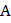# A particlemoves with constant speed on a circle in anticlockwise direction as shown in figureMatch column I and with Column II: Column-I Column- II (A) Angular momentum of the particle about(p) Is minimum when the particle is at(B) Angular momentum of the particle about(q) Is maximum when the particle is at(C) Angular velocity of the particle about(r) Does not remain constant (D) Angular velocity of the particle about(s) Remains constant CODES :   A B C D     a) D b,c d a,c     b) b c d a     c) a b c d     d) b,a c d a

## Question ID - 100968 :- A particlemoves with constant speed on a circle in anticlockwise direction as shown in figureMatch column I and with Column II: Column-I Column- II (A) Angular momentum of the particle about(p) Is minimum when the particle is at(B) Angular momentum of the particle about(q) Is maximum when the particle is at(C) Angular velocity of the particle about(r) Does not remain constant (D) Angular velocity of the particle about(s) Remains constant CODES :   A B C D     a) D b,c d a,c     b) b c d a     c) a b c d     d) b,a c d a

3537

 (a) We know that angular momentum= linear momentumperpendicular distance. So aboutangular momumtum will remain constant. But aboutit will keep on changing. It is maximum aboutwhen the particle is at, because then the perpendicular distance is maximum We know that angular velocity. So aboutangular velocity will remain constant. But aboutit will keep on changing. It is a minimum aboutwhen the particle is at, because then the perpendicular distanceis maximum

Next Question :

Complete the given number/letter analogy, by choosing the correct answer from the given alternatives.

 V B R M T H : E O I Z G U : : P F X L W A : ? (a) K S C Y D N (b) K S X C W N (c) K F C Y W Z (d) K F X C D Z